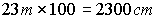Conversion Tables and Methods to Use when converting

from Metric Units to Metric Units (Length)

Some terms to remember

 Meter – Length Kilo – Thousand Liter – Volume Milli – Thousand Gram – Mass/Weight Centi – Hundred Celsius – Temperature Deci – Ten

### Measure of Lengths

 10 millimeters (mm) = 1 centimeter (cm) 10 centimeters = 1 decimeter (dm) = 100 millimeters 100 centimeter = 1 meter (m) = 1,000 millimeters 1000 meters = 1 kilometer (km)

### Operations for Length

Example: To get Centimeters from Meters you multiply the Meters by 100

You Have: 23 Meters

You want Centimters: So, Centimeters = 23 Meters

We Multiply the meters by 100:You want Centimters: So, Centimeters = 23 Meters

There are 2300 centimeters in 23 meters or 100 centimeters for every meter.

 If you have this Do this To get this millimeters (mm) Divide by 10 (mm/10) centimeter (cm) centimeters (cm) Multiply by 10 (cm * 10) Millimeters (mm) Meters (m) Multiply by 100 (m * 100) Centimeters (cm) Centimeters (cm) Divide by 100 (cm/100) Meters (m) Millimeters (mm) Divide by 1000 (mm/1000) Meters (m)
[page 1] [page 2] [page 3] [page 4] [page 5] [page 6] [page 7] [evaluation]

https://mste.illinois.edu/dildine/tcd_files/program15.htm